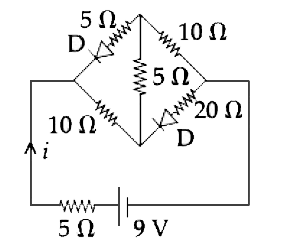Question

Updated on:26/04/2023

# The current I in the network is :Text Solution

A
0.6Å
B
0.2Å
C
0.3Å
D
0Å

Transcript

hello Jivan the question is that the current I in the network is this question I have to find out the value of current I now it is given so this post to Terminal is connected with this diode with entertainment and hence both side will be reversed by Sudhir diode reverse bias Suno current will flow through this branch and similarly this register is diode is also reverse bias Suno can flow through this 500 current will flow only through this path sunao I can draw the circuit gain 10 ohm resistance 5 ohm resistance and this is \$10 system and of 5 ohm resistance is outside and a battery of 9 volt is there a current is flowing through the circuit so these values then know this value is 5 and this values then no 5 now resistance will be so I will be equal to both all resistance in series so this will became 10 + 5 +
plus 5 10 + 10 2020 + 10 that will be equal to 30 ohm and batteries give so it is given value as a 9 volt so according to Ohm's law current through the circuit current through the circuit will be = 2y = to wear on our value with 9 volt and value of RS 30 now sold this value so value current will be = 2.3 a health this is our items were so this is value of 4.3 ampere no thanks from sorry for this Christmas painting Subah 2.3 ohm is a writers with thank you